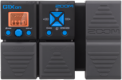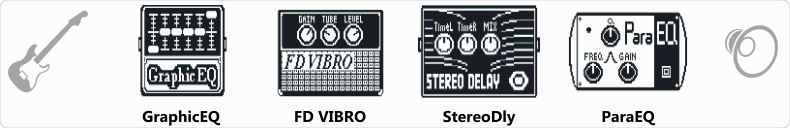Discussion in 'Zoom G1on/G1Xon' started by Kovács László, Feb 3, 2021.Device: Zoom G1Xon
Firmware: 1.21

Optimized for: Phones/Speaker

Effects chain:Shadows patch for home. Direct to soundcard.

Effect: "GraphicEQ" (Dynamics / Filter), active - "yes"
"160Hz" = 0
"400Hz" = 0
"800Hz" = -3
"3.2kHz" = 8
"6.4kHz" = -3
"12kHz" = -4
"Level" = 150

Effect: "FD VIBRO" (Amp simulators), active - "yes"
"Gain" = 12
"Tube" = 27
"Level" = 112
"Trebl" = 36
"Middl" = 93
"Bass" = 47
"Prese" = 0
"CAB" = ALIEN 4x12
"OUT" = LINE

Effect: "StereoDly" (Delay / Reverb), active - "yes"
"TimeL" = 126
"TimeR" = 458
"Mix" = 85
"LchFB" = 20
"RchFB" = 34
"Level" = 90
"LchLv" = 99
"RchLv" = 85
"Tail" = Off

Effect: "ParaEQ" (Dynamics / Filter), active - "yes"
"Freq1" = 250Hz
"Q1" = 0.5
"Gain1" = 6
"Freq2" = 2.5kHz
"Q2" = 1
"Gain2" = 10
"Level" = 150
Patch Volume: 100

Note: This is a patch file, you will need to download and install the ToneLib-Zoom software to use the patch.